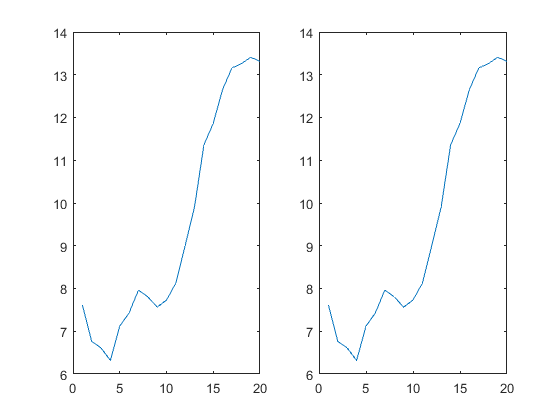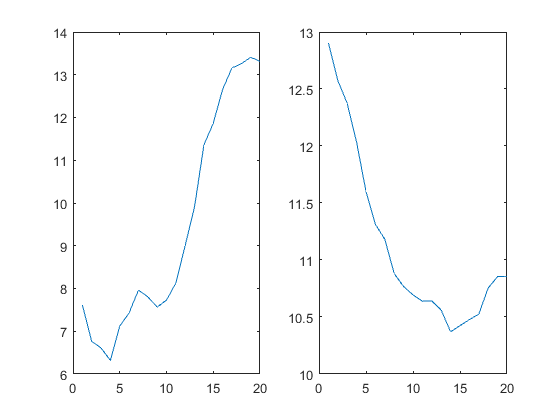Hello，everyone！

# 1 理论

Procrustes Analysis普氏分析法，此博客对普氏分析法理论介绍的还不错，有兴趣的同学可以读一读。

# 2 MATLAB函数

## 2.1 MATLAB函数介绍-procrustes

 procrustes Procrustes Analysis
D = procrustes(X, Y) determines a linear transformation (translation,
reflection, orthogonal rotation, and scaling) of the points in the
matrix Y to best conform them to the points in the matrix X.  The
"goodness-of-fit" criterion is the sum of squared errors.  procrustes
returns the minimized value of this dissimilarity measure in D.  D is
standardized by a measure of the scale of X, given by

sum(sum((X - repmat(mean(X,1), size(X,1), 1)).^2, 1))

i.e., the sum of squared elements of a centered version of X.  However,
if X comprises repetitions of the same point, the sum of squared errors
is not standardized.

X and Y are assumed to have the same number of points (rows), and
procrustes matches the i'th point in Y to the i'th point in X.  Points
in Y can have smaller dimension (number of columns) than those in X.
In this case, procrustes adds columns of zeros to Y as necessary.

[D, Z] = procrustes(X, Y) also returns the transformed Y values.

[D, Z, TRANSFORM] = procrustes(X, Y) also returns the transformation
that maps Y to Z.  TRANSFORM is a structure with fields:
c:  the translation component
T:  the orthogonal rotation and reflection component
b:  the scale component
That is, Z = TRANSFORM.b * Y * TRANSFORM.T + TRANSFORM.c.

[...] = procrustes(..., 'Scaling',false) computes a procrustes solution
that does not include a scale component, that is, TRANSFORM.b == 1.
procrustes(..., 'Scaling',true) computes a procrustes solution that
does include a scale component, which is the default.

[...] = procrustes(..., 'Reflection',false) computes a procrustes solution
that does not include a reflection component, that is, DET(TRANSFORM.T) is
1.  procrustes(..., 'Reflection','best') computes the best fit procrustes
solution, which may or may not include a reflection component, 'best' is
the default.  procrustes(..., 'Reflection',true) forces the solution to
include a reflection component, that is, DET(TRANSFORM.T) is -1.

Examples:

% Create some random points in two dimensions
n = 10;
X = normrnd(0, 1, [n 2]);

% Those same points, rotated, scaled, translated, plus some noise
S = [0.5 -sqrt(3)/2; sqrt(3)/2 0.5]; % rotate 60 degrees
Y = normrnd(0.5*X*S + 2, 0.05, n, 2);

% Conform Y to X, plot original X and Y, and transformed Y
[d, Z, tr] = procrustes(X,Y);
plot(X(:,1),X(:,2),'rx', Y(:,1),Y(:,2),'b.', Z(:,1),Z(:,2),'bx');


## 2.2 procrustes 测试实例

1. data 数据集
data =
7.61597019000000
6.76320399000000
6.60918327200000
6.31725582000000
7.11964454600000
7.43044326800000
7.96325953100000
7.80692535700000
7.56442238700000
7.72914905000000
8.12539049300000
9.00709395200000
9.90960423500000
11.3552868200000
11.8654178800000
12.6554510100000
13.1595179900000
13.2571674800000
13.4085209200000
13.3154797800000
12.9042477900000
12.5759433300000
12.3723241500000
12.0329882400000
11.6008698000000
11.3132455600000
11.1818076300000
10.8807610100000
10.7673431100000
10.6941123700000
10.6389603000000
10.6410223100000
10.5608535500000
10.3697213600000
10.4243787300000
10.4770807500000
10.5255527700000
10.7548124400000
10.8544539000000
10.8575230400000

1. matlab代码
% 图像差异
figure
subplot(1,2,1)
plot(data(1:20));
subplot(1,2,2)
plot(data(1:20));
figure
subplot(1,2,1)
plot(data(1:20));
subplot(1,2,2)
plot(data(21:40));
% 结果差异
procrustes(data(1:20),data(1:20))
procrustes(data(1:20),data(21:40))

1. 仿真结果
• 图片• 结果
ans =

0

ans =

0.616312-27744
03-19249
11-151万+
11-065566
12-091万+
08-066702
06-195184
02-011万+
12-21894
12-062647
07-287375
04-219908
08-215508
01-259960
07-058890
08-045745